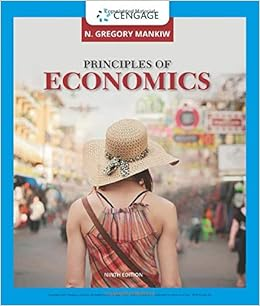# When the output gap is negative the actual

• Test Prep
• 42
• 90% (49) 44 out of 49 people found this document helpful

This preview shows page 35 - 38 out of 42 pages.

##### We have textbook solutions for you!
The document you are viewing contains questions related to this textbook.The document you are viewing contains questions related to this textbook.
Chapter 35 / Exercise 1
Principles of Economics
MankiwExpert Verified
10.When the output gap is negative, the actual unemployment rate is:A)above the natural rate.B)below the natural rate.C)equal to the natural rate.D)The actual and natural unemployment rates arenot related to the output gap.
11.When the output gap is _________, reflecting an inflationary gap, the unemployment rate is _________ the natural rate of unemployment.
12.The natural rate of unemployment is 4%, and the economy is producing 95% of its potential output. Okun's law predicts an unemployment rate of:
13.According to the short-run Phillips curve, when actual real GDP is _________ potential output, the price level _________ and the unemployment rate falls.
##### We have textbook solutions for you!
The document you are viewing contains questions related to this textbook.The document you are viewing contains questions related to this textbook.
Chapter 35 / Exercise 1
Principles of Economics
MankiwExpert Verified
14.The short-run Phillips curve represents the relationship between the unemployment rate and the rate of change in:A)the interest rate.B)output.C)wages only.D)the aggregate price level.
15.Suppose that the unemployment rate rises at the same time that the inflation rate declines. This situation would be consistent with a movement along the:
16.Suppose you are told that the short-run Phillips curve has shifted downward. Which of the following must have happened?
17.If workers expect a lower rate of inflation, the short-run Phillips curve will:
18.Which of the following accurately describes disinflation?A)It must be accompanied by a decline in the price level.B)The inflation rate rises at a higher rate.C)It is the process of bringing down the inflation that has become embedded in expectations.D)It is a gradual reduction in the price level over time.
19.The cost of disinflation is the:
20. Deflation:
Chapter 19
•••